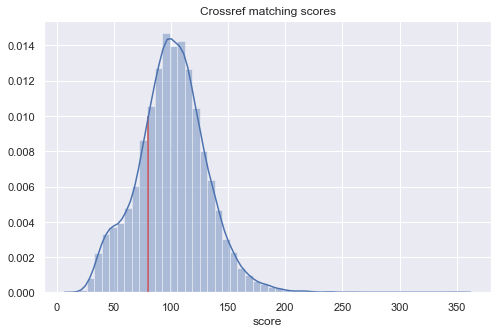# Progress report 1¶

Asura Enkhbayar, 09.03.2020

This report covers intermediate results for:

• Citation parsing on the unstructured references in order to retrieve identifiers and other metadata in the input dataset
• Crossref queries using the unstructured references from the original dataset
In [ ]:
import numpy as np
import os
from pathlib import Path

import matplotlib.pyplot as plt
import pandas as pd
import seaborn as sns

from tracking_grants import project_dir, data_dir
from tracking_grants import CR_THRESH

In [ ]:
# Articles after processing with anystyle
articles = pd.read_csv(data_dir / "interim/structured.csv", index_col="article_id")

# External data from CR/Altmetric
crossref = pd.read_csv(data_dir / "interim/_crossref.csv", index_col="article_id", low_memory=False)
altmetric = pd.read_csv(data_dir / "interim/_altmetric.csv", index_col="article_id", low_memory=False)
ncbi = pd.read_csv(data_dir / "interim/_ncbi.csv", index_col="article_id")


## Citation Parsing¶

Our input dataset contains unstructured references in the form of strings that were typed in by the original authors.

Using anystyle we can attempt to retrieve DOIs, PMIDs, PMCIDs, and other structured metadata from these strings.

In :
x = articles[['DOI', 'PMID', 'PMCID']].count().to_frame('Articles with')
x[f'% (n={len(articles)})'] = 100 * x['Articles with'] / len(articles)
x.round(1)

Out:
Articles with % (n=18708)
DOI 546 2.9
PMID 163 0.9
PMCID 96 0.5

As we can see the number of identifiers extractred from the input dataset is not really useful.

## Crossref results¶

Crossref provides an API endpoint for textual searches using references. We are using that endpoint and retrieving the best candidate for each query. The results contain a score which refers to the quality of the match.

We are currently using 80 as the threshold for that score. We are still hoping to get in touch with one developer at Crossref who has been working on citation matching.

In :
CR_THRESH

Out:
80

The following plot shows the distribution of matching scores including the score of 80 which I have currently chosen based on some prelim experimentation and manual inspection of random articles.

In :
sns.distplot(crossref.score)
plt.title("Crossref matching scores")
plt.vlines(cr_thresh, 0, 0.010, "r");In :
# Join article metadata and crossref results
df = articles[['type', 'DOI', 'PMCID', 'PMID']]
df = df.join(crossref, rsuffix="_cr")


Different thresholds and the resulting number of matches:

In :
scores = df.score
ts = [60, 70, 80, 90, 100]

for t in ts:
print(f"{t}: {scores.where(scores>=t).count()} articles ({100*scores.where(scores>=t).count()//len(articles)}%)")

60: 16906 articles (90%)
70: 15934 articles (85%)
80: 14406 articles (77%)
90: 12248 articles (65%)
100: 9594 articles (51%)


For this particular notebook, I am using the threshold of 80 to determine which articles were found in Crossref.

In :
# Filter out DOIs that had a score lower than 80
df.loc[df.score < cr_thresh, 'DOI_cr'] = None
articles_with_doi = df[df.DOI_cr.notna()].index

In :
x = df[['DOI_cr']].count().to_frame('Articles with')
x[f'% (n={len(articles)})'] = 100 * x['Articles with'] / len(articles)
x.round(1)

Out:
Articles with % (n=18708)
DOI_cr 14406 77.0

Using a minimum score of 80, we have found 14,406 DOIs in Crossref which corresponds to 77% of the original dataset

Using the APIs provided by the NCBI we can now also attempt to convert DOIs to pmid/pmcid.

In :
x = ncbi[['pmid', 'pmcid']].count().to_frame('Articles with')
x[f'% (n={len(articles)})'] = 100 * x['Articles with'] / len(articles)
x.round(1)

Out:
Articles with % (n=18708)
pmid 5112 27.3
pmcid 5123 27.4

The results for the NCBI API were not very great once I started to manually check several examples. Furthermore, we can only retrieve pmid/pmcids for articles that already have a DOI. These identifiers are therefore not really useful for the processing pipeline, but might be interesting to report nevertheless.

## Altmetric results¶

We can use these DOIs to retrieve altmetrics for these articles.

In :
df2 = altmetric.reindex(articles_with_doi)
df2 = df2[['altmetric_id', 'cited_by_tweeters_count', 'cited_by_fbwalls_count',
'cited_by_feeds_count', 'cited_by_msm_count', 'cited_by_wikipedia_count', 'cited_by_rdts_count']]
df2.columns = ["altmetric_id", 'tweets', 'fb_mentions', 'blogposts', 'news', 'wikipedia', 'reddit']


### Coverage¶

First percentage only considers articles that had a DOI (n=14,406)

Second percentage uses the input dataset as the denominator (n=18,708)

In :
x = df2.count().to_frame('Articles with')
x[f'% (n={len(df2)})'] = 100 * x['Articles with'] / len(df2)
x.round(1).sort_values("Articles with", ascending=False)

Out:
Articles with % (n=14406)
altmetric_id 7117 49.4
tweets 2126 14.8
wikipedia 1171 8.1
fb_mentions 609 4.2
news 541 3.8
blogposts 520 3.6
reddit 37 0.3
• 7,117 articles (50% of the articles with DOI) returned with an altmetric_id
• 8.1% for Wikipedia (is that considered high?)

### Details for altmetric counts¶

In :
df2.describe().round(1)

Out:
altmetric_id tweets fb_mentions blogposts news wikipedia reddit
count 7117.0 2126.0 609.0 520.0 541.0 1171.0 37.0
mean 18811159.0 7.9 4.6 1.6 5.0 1.4 1.3
std 18072344.1 24.5 62.3 1.5 9.0 1.7 0.7
min 101417.0 1.0 1.0 1.0 1.0 1.0 1.0
25% 3311373.0 1.0 1.0 1.0 1.0 1.0 1.0
50% 8181500.0 2.0 1.0 1.0 2.0 1.0 1.0
75% 41322904.0 6.0 2.0 2.0 6.0 1.0 1.0
max 76583497.0 460.0 1538.0 18.0 114.0 36.0 4.0